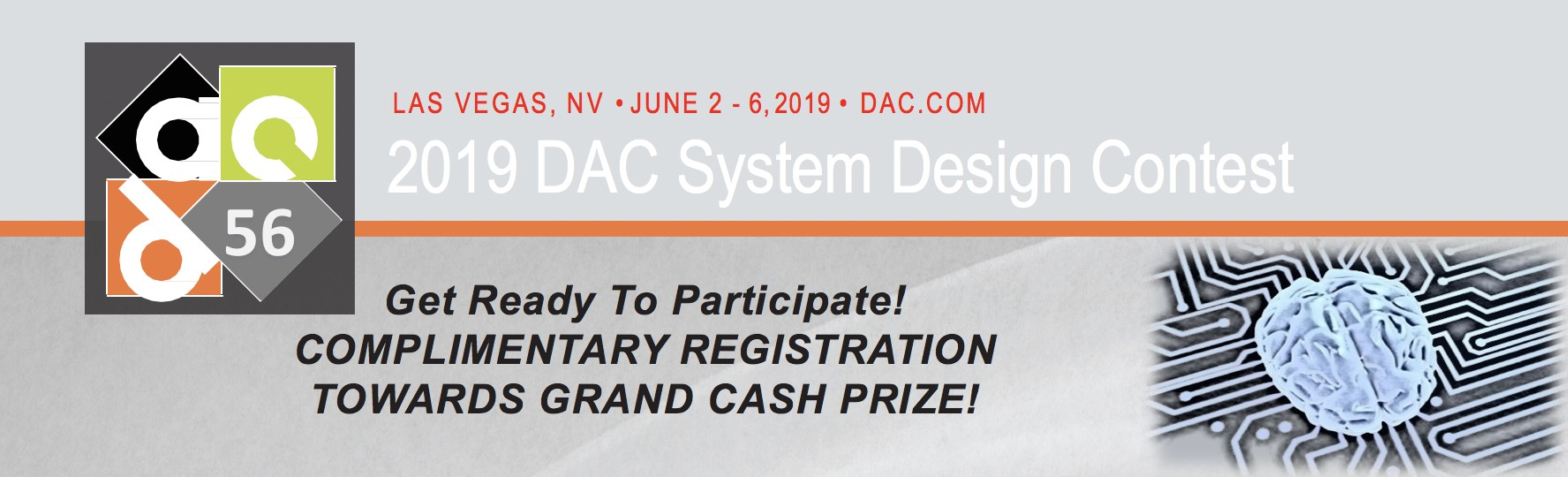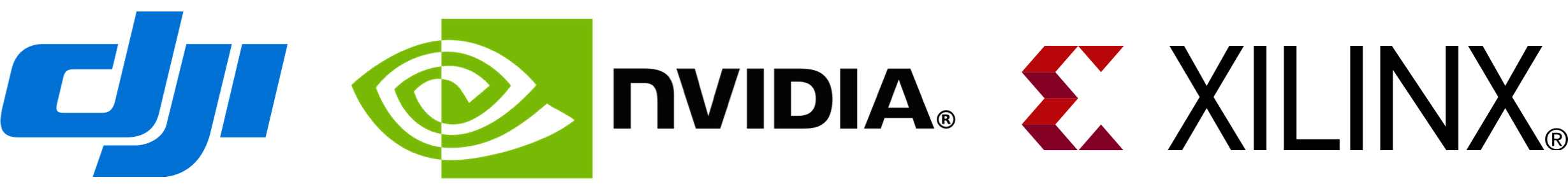# 2019 DAC System Design Contest

 Contest announcement: October 18, 2018 Registration deadline: November 30, 2018 Host webinars December 10, 2018 Contest closes: May, 2019 Award presentation: DAC 2019

Award: grand cash prize for the top three teams.

## Registration

Each team is required to register at the following link: Registration Link.

## Overview:

The 2019 System Design Contest features embedded system implementation of neural network based object detection for drones. Contestants will receive training dataset provided by our industry sponsor DJI, and a hidden dataset will be used to evaluate the performance of the designs in terms of accuracy and power. Contestants will compete in two different categories: FPGA and GPU, and grand cash awards will be given to the top three teams in each category. In addition, our industry sponsor Xilinx and Nvidia will provide a limited number of teams successfully registered with a free design kit (on a first-come-first-served basis). The award ceremony will be held at 2019 IEEE/ACM Design Automation Conference.

#### Competition:

Contestants are required to select one of the target platforms to exploit machine learning algorithms for the chosen application.

#### Eligibility:

The contest is open to both industry and academia.

## Target Platforms

• Nvidia Jetson TX2

• Xilinx Ultra 96

## Design resource:Please click this link for The 2018 DAC SDC winners design entry.

## Submission:

Each team will submit their design once each month until the final deadline, and the ranking will be updated monthly. Following are detailed submission guideline:

In submission, please use the following XML format for the output:

<annotation>
<filename>0001</filename>
<size>
<width>640</width>
<height>360</height>
</size>
<object>
<bndbox>
<xmin>300</xmin>
<ymin>154</ymin>
<xmax>355</xmax>
<ymax>210</ymax>
</bndbox>
</object>
</annotation>


## Evaluation:

The evaluation for the design is based on the accuracy, throughput, and energy consumption.

• Intersection over Union (IoU) for object detection: Intersection over Union is an evaluation metric used to measure the accuracy of an object detector on a particular dataset. Note that we only care the IoU results, but do NOT care the classification result.

• Throughput: The minimum speed requirement (20FPS on GPU and 10FPS on FPGA) in this competition has to be met. If the FPS is lower than the requirement, then a penalty to IoU will occur: IoU(real) = IoU(measure) * [ min[FPS(measure), requirement]/requirement].

• Energy: Energy consumption for a detector to process all the images.

Formally, to apply IoU to evaluate an object detector we need:

• The ground-truth bounding boxes, denoted by $$GroundTruth$$ (i.e., the labeled bounding boxes that specify where in the image the object is in the xml files).

• The detected bounding boxes from the model, denoted by $$DetectionResult$$.

Suppose we have $$I$$ registered teams (models); the dataset contains $$K$$ evaluation images. Let $$IoU_{i_k}$$ be the IoU score of image $$k (k \le K)$$ for team $$i (i \le I)$$. It is computed by:

$IoU_{i_k} = \cfrac{\text{Area of Overlap}}{\text{Area of Union}} = \cfrac{DetectionResult \cap GroundTruth}{DetectionResult \cup GroundTruth}.$

A good example of Intersection over Union can be found at here.

Let $$R_{IoU_i}$$ be the IoU score for team $$i$$. It is computed as

$R_{IoU_i} = \cfrac{\sum_{k=1}^K IoU_{i_k}}{K}.$

Let $$E_i$$ be the energy consumption of processing all $$K$$ images for team $$i$$. Let $$\bar{E_I}$$ be the average energy consumption of $$I$$ teams. It is computed as

$\bar{E_I} = \cfrac{\sum_{i=1}^I E_i}{I}.$

Let $$ES_i$$ be the energy consumption score for team $$i$$. It is computed as

$ES_i = \max\{0, 1 + 0.2 \times \log_x \cfrac{\bar{E_I}}{E_i} \},$

where x is 2 for FPGA platform and 10 for GPU platform. Let $$TS_i$$ be the total score for team $$i$$, which is computed by

$TS_i = R_{IoU_i} \times (1 + ES_i),$

• $$I$$: total number of registered teams

• $$i$$: index of a team among all teams

• $$K$$: total number of images in the dataset

• $$k$$: index of an image in the dataset

Note: The dataset provided for participants to download contains 70% of the total dataset provided by our sponsor. The remaining 30% of the dataset is reserved for our evaluation. We will ONLY use the reserved dataset to evaluate and rank all the teams.

## References:

The works below addresses object detection in an end-to-end manner. They have simple pipelines, can work in real-time and are suitable for system implementation. Also, they provide source codes and deep learning models and may be used as a good starting point.

• David Held, Sebastian Thrun, et al. “Learning to Track at 100 FPS with Deep Regression Networks

• Milan, A. and Rezatofighi, et al. “Online Multi-Target Tracking with Recurrent Neural Networks

• Luca Bertinetto, Jack Valmadre, et al. “Fully-Convolutional Siamese Networks for Object Tracking

• Joseph Redmon, Santosh Divvala, et al. “You Only Look Once: Unified, Real-Time Object Detection

• Wei Liu, Dragomir Anguelov, et al. “SSD: Single Shot MultiBox Detector

## Contest Organizers:

 Jingtong Hu University of Pittsburgh Jeff Goeders Brigham Young University Phillip Brisk University of California, Riverside Yanzhi Wang Northeastern University Guojie Luo Peking University Chris Rowen Cognite Ventures Bei Yu Chinese University of Hong Kong Naveen Purushotham Xilinx